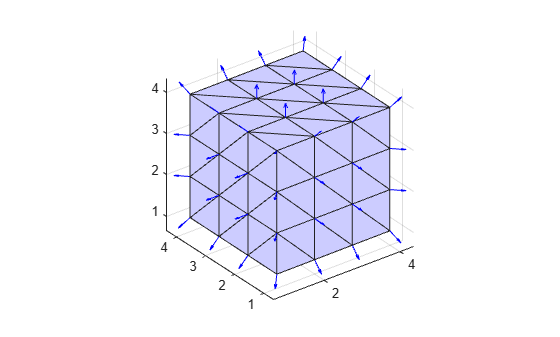# vertexNormal

Triangulation vertex normal

## Syntax

``V = vertexNormal(TR)``
``V = vertexNormal(TR,ID)``

## Description

example

````V = vertexNormal(TR)` returns the unit normal vectors to all vertices in a 3-D surface triangulation. `V` is a three-column matrix with each row containing the unit normal coordinates corresponding to the vertices in `TR.Points`.```
````V = vertexNormal(TR,ID)` returns the unit normal vector to each vertex indexed by `ID`. A vertex ID is the row number corresponding to the vertices in the property `TR.Points`.```

## Examples

collapse all

Compute and plot the unit normal vectors to the vertices of a triangulation.

Create a 3-D triangulation representing the volume of a cube.

```[X,Y,Z] = meshgrid(1:4); x = X(:); y = Y(:); z = Z(:); DT = delaunayTriangulation(x,y,z);```

Triangulate the boundary of the cube.

```[Tfb,Xfb] = freeBoundary(DT); TR = triangulation(Tfb,Xfb);```

Find the unit normal vectors to the triangle vertices.

`V = vertexNormal(TR);`

Plot the triangulated surface and the unit normal vectors.

```trisurf(TR,'FaceColor',[0.8 0.8 1.0]); axis equal hold on quiver3(Xfb(:,1),Xfb(:,2),Xfb(:,3), ... V(:,1),V(:,2),V(:,3),0.5,'Color','b');```## Input Arguments

collapse all

Triangulation representation for 3-D surface triangulations only, specified as a scalar `triangulation` or `delaunayTriangulation` object.

Data Types: `triangulation` | `delaunayTriangulation`

Vertex IDs, specified as a scalar or a column vector whose elements correspond to a single vertex in the triangulation object. The ID of each vertex is the corresponding row number of the vertices in the `Points` property.

Data Types: `double`

## Version History

Introduced in R2013a